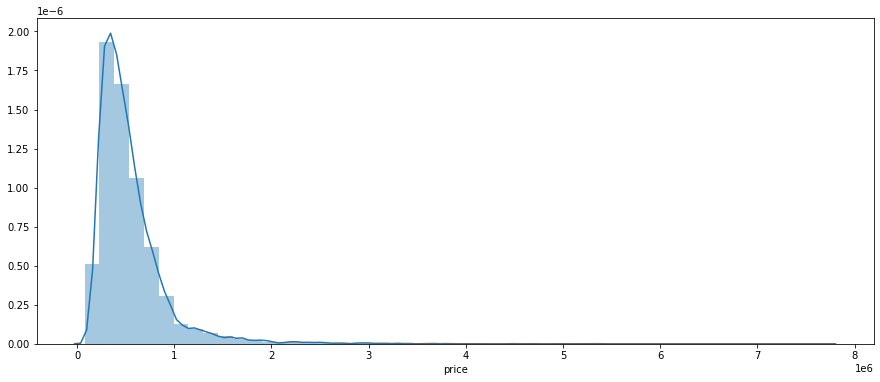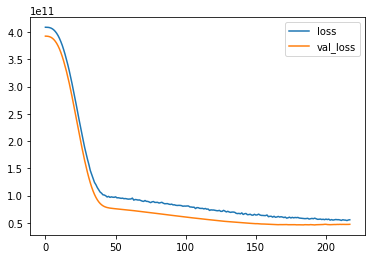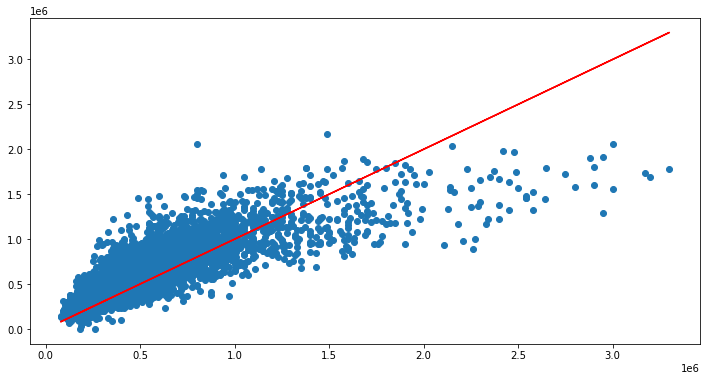This is a more complex example of Keras, utilizing Regression. This utilizes a good sized dataset from Kaggle, but does contain a little bit of data cleansing before we can build out the model. Unfortunately the model we end up building isn’t perfect and requires more tuning or some final dataset alterations, but it’s a good example none the less. More information below.

## Keras / Tensorflow Regression – Example

Here we’re going to attempt to utilize Keras/Tensorflow to predict the price of homes based upon a set of features.

The data being used comes from Kaggle:

https://www.kaggle.com/harlfoxem/housesalesprediction

### Imports

In :
```import pandas as pd
import numpy as np
import matplotlib.pyplot as plt
import seaborn as sns
```

### Data Exploration and Cleansing

In :
```df = pd.read_csv('DATA/kc_house_data.csv')
```

Since we’re going to predict prices, we can do a quick distribution. We can see the vast majority sit around 500k, and we have some outliers all the way out to 7m+ (but very few).

In :
```plt.figure(figsize=(15,6))
sns.distplot(df['price'])
```
Out:
`<AxesSubplot:xlabel='price'>`One thing we may want to do is get rid of these outliers (at least to an extent). These will certainly affect our model and may skew results. Sorting by price (and from the visual above) we can see that ~3.5m may be a logical cutoff for keeping data.

In :
```df.sort_values('price',ascending=False).head(20)
```
Out:
id date price bedrooms bathrooms sqft_living sqft_lot floors waterfront view grade sqft_above sqft_basement yr_built yr_renovated zipcode lat long sqft_living15 sqft_lot15
7245 6762700020 10/13/2014 7700000.0 6 8.00 12050 27600 2.5 0 3 13 8570 3480 1910 1987 98102 47.6298 -122.323 3940 8800
3910 9808700762 6/11/2014 7060000.0 5 4.50 10040 37325 2.0 1 2 11 7680 2360 1940 2001 98004 47.6500 -122.214 3930 25449
9245 9208900037 9/19/2014 6890000.0 6 7.75 9890 31374 2.0 0 4 13 8860 1030 2001 0 98039 47.6305 -122.240 4540 42730
4407 2470100110 8/4/2014 5570000.0 5 5.75 9200 35069 2.0 0 0 13 6200 3000 2001 0 98039 47.6289 -122.233 3560 24345
1446 8907500070 4/13/2015 5350000.0 5 5.00 8000 23985 2.0 0 4 12 6720 1280 2009 0 98004 47.6232 -122.220 4600 21750
1313 7558700030 4/13/2015 5300000.0 6 6.00 7390 24829 2.0 1 4 12 5000 2390 1991 0 98040 47.5631 -122.210 4320 24619
1162 1247600105 10/20/2014 5110000.0 5 5.25 8010 45517 2.0 1 4 12 5990 2020 1999 0 98033 47.6767 -122.211 3430 26788
8085 1924059029 6/17/2014 4670000.0 5 6.75 9640 13068 1.0 1 4 12 4820 4820 1983 2009 98040 47.5570 -122.210 3270 10454
2624 7738500731 8/15/2014 4500000.0 5 5.50 6640 40014 2.0 1 4 12 6350 290 2004 0 98155 47.7493 -122.280 3030 23408
8629 3835500195 6/18/2014 4490000.0 4 3.00 6430 27517 2.0 0 0 12 6430 0 2001 0 98004 47.6208 -122.219 3720 14592
12358 6065300370 5/6/2015 4210000.0 5 6.00 7440 21540 2.0 0 0 12 5550 1890 2003 0 98006 47.5692 -122.189 4740 19329
4145 6447300265 10/14/2014 4000000.0 4 5.50 7080 16573 2.0 0 0 12 5760 1320 2008 0 98039 47.6151 -122.224 3140 15996
2083 8106100105 11/14/2014 3850000.0 4 4.25 5770 21300 2.0 1 4 11 5770 0 1980 0 98040 47.5850 -122.222 4620 22748
7028 853200010 7/1/2014 3800000.0 5 5.50 7050 42840 1.0 0 2 13 4320 2730 1978 0 98004 47.6229 -122.220 5070 20570
19002 2303900100 9/11/2014 3800000.0 3 4.25 5510 35000 2.0 0 4 13 4910 600 1997 0 98177 47.7296 -122.370 3430 45302
16288 7397300170 5/30/2014 3710000.0 4 3.50 5550 28078 2.0 0 2 12 3350 2200 2000 0 98039 47.6395 -122.234 2980 19602
18467 4389201095 5/11/2015 3650000.0 5 3.75 5020 8694 2.0 0 1 12 3970 1050 2007 0 98004 47.6146 -122.213 4190 11275
6502 4217402115 4/21/2015 3650000.0 6 4.75 5480 19401 1.5 1 4 11 3910 1570 1936 0 98105 47.6515 -122.277 3510 15810
15241 2425049063 9/11/2014 3640000.0 4 3.25 4830 22257 2.0 1 4 11 4830 0 1990 0 98039 47.6409 -122.241 3820 25582
19133 3625049042 10/11/2014 3640000.0 5 6.00 5490 19897 2.0 0 0 12 5490 0 2005 0 98039 47.6165 -122.236 2910 17600

20 rows Ã— 21 columns

If we take this concept, we can find out what percentage of homes are above 350k

In :
```#Original
df.shape
```
Out:
`(21597, 21)`
In :
```#Above 350k
df[df['price'] <= 3500000].shape
```
Out:
`(21575, 21)`

This accounts for less than a percentage of homes, so we could just kick these out completely.

In :
```#Kick out outliers
df = df[df['price'] <= 3500000]
```

We do have a date column in the DataFrame, but it’ll probably make more sense to convert these to month/year to allow for better analysis

In :
```#Convert string to date
df['date'] = pd.to_datetime(df['date'])

df['year'] = df['date'].apply(lambda date : date.year)
df['month'] = df['date'].apply(lambda date : date.month)
```
In :
```#Drop since we no longer need the original field
df = df.drop('date',axis=1)
```

In addition – we can drop a few other fields we won’t need. ID will not serve any purpose in our model and can be thrown away. In addition, Zipcode is really not in a useful format for predictive analysis and would need to be transformed in some way. However, in reading up on this dataset – folks seem to agree that this data seems bad or incorrect. So, I’m going to cut my losses and also toss it.

In :
```df = df.drop('id',axis=1)
df = df.drop('zipcode',axis=1)
```
In :
```df.head()
```
Out:
price bedrooms bathrooms sqft_living sqft_lot floors waterfront view condition grade sqft_above sqft_basement yr_built yr_renovated lat long sqft_living15 sqft_lot15 year month
0 221900.0 3 1.00 1180 5650 1.0 0 0 3 7 1180 0 1955 0 47.5112 -122.257 1340 5650 2014 10
1 538000.0 3 2.25 2570 7242 2.0 0 0 3 7 2170 400 1951 1991 47.7210 -122.319 1690 7639 2014 12
2 180000.0 2 1.00 770 10000 1.0 0 0 3 6 770 0 1933 0 47.7379 -122.233 2720 8062 2015 2
3 604000.0 4 3.00 1960 5000 1.0 0 0 5 7 1050 910 1965 0 47.5208 -122.393 1360 5000 2014 12
4 510000.0 3 2.00 1680 8080 1.0 0 0 3 8 1680 0 1987 0 47.6168 -122.045 1800 7503 2015 2

### Build Model

In :
```#Separate features from label
X = df.drop('price',axis=1)
y = df['price']
```
In :
```from sklearn.model_selection import train_test_split
```
In :
```#Perform splitting
X_train, X_test, y_train, y_test = train_test_split(X, y, test_size=0.3, random_state=101)
```

#### Perform Scaling

Since the data across all fields varies, it’s best to scale it first

In :
```from sklearn.preprocessing import MinMaxScaler
```
In :
```scaler = MinMaxScaler()
```
In :
```#Fit and transform training and test set
X_train = scaler.fit_transform(X_train)
X_test = scaler.fit_transform(X_test)
```

#### Fit to Neural Network

In :
```from tensorflow.keras.models import Sequential
from tensorflow.keras.layers import Dense
from tensorflow.keras.layers import Dropout
```
In :
```#Try to base model off size of dataset
X_train.shape
```
Out:
`(15102, 19)`
In :
```model = Sequential()

#Make it 19 neurons since we have 19 cols
model.add(Dropout(0.25)) #Choose somewhere between 0/1 (1 = 100%) of neurons to turn off randomly to avoid overfitting

```
In :
```from tensorflow.keras.callbacks import EarlyStopping
early_stop = EarlyStopping(monitor='val_loss',mode='min',verbose=1,patience=25)
```

In an attempt to see how our model actual performs (and if we’re overfitting), we’re going to utilize the validation_data parameter to pass in the test values, so we can compare the predictions against actual. A batch_size of 128 should help to avoid overfitting (smaller should be better here). I’ve also implemented a dropout of 25% on the layers, and an early stop in case overfitting is detected.

In :
```model.fit(x=X_train,y=y_train.values,
validation_data=(X_test,y_test.values),
batch_size=128,epochs=400,callbacks=[early_stop])
```

Compare the losses of the training vs. test dataset to see if we’re overfitting.

If you see the orange line (val_loss) go up towards the end it means your overfitting, because now you have a much larger loss on your validation data.

With the plot below, we do see the val_loss start to slightly rise above 200 epochs which could indicate a little bit of overfitting with the data. The early stopping caught this and stopped us from going the entire 400 epochs

In :
```losses = pd.DataFrame(model.history.history)
losses.plot()
```
Out:
`<AxesSubplot:>`#### Predict and Evaluate

In :
```from sklearn.metrics import mean_squared_error,mean_absolute_error,explained_variance_score
```
In :
```#Grab predictions from the testing set
predictions = model.predict(X_test)
```
In :
```#MAE
mean_absolute_error(y_test,predictions)
```
Out:
`158519.56384873268`
In :
```#Mean price
df['price'].mean()
```
Out:
`536130.9431286211`

The Mean Absolute Error indicates we’re off by an average of 158k, which isn’t great given we’re looking at an average home price of ~536k. Meaning we’re off a little under 30%. I’m guessing we’re still encountering too much interference from outliers above 350k – so let’s continue.

In :
```#MSE
np.sqrt(mean_squared_error(y_test,predictions))
```
Out:
`217338.48768344492`
In :
```#Best possible score is 1.0
#How much variance is explained by our model?
explained_variance_score(y_test,predictions)
```
Out:
`0.6452589976965329`

You can see we’re only explaining approximately 64% of variance in the data. We could try to plot this data out to see if can visually see how well we’re actually doing.

In :
```plt.figure(figsize=(12,6))
plt.scatter(y_test,predictions)
plt.plot(y_test,y_test,'r')
```
Out:
`[<matplotlib.lines.Line2D at 0x172f6d92c88>]`We’re actually doing a good job on predictions on prices below 1.5m or so. The problem seems to exist for the more expensive houses.

### Predict New Home Prices

So how can we predict the price of a net new home? In the example below we take the first house out of the DataFrame and remove the price. Then we scale the data and predict using our trained model. Now we can compare the price predicted against the actual.

In :
```#Assign the first house in the DataFrame to a new DataFrame
single_house = df.drop('price',axis=1).iloc
```
In :
```#Scale the values AND reshape the results (so the shape matches the expected shape for the model)
single_house = scaler.transform(single_house.values.reshape(-1,19))
```
In :
```#Predict the price
model.predict(single_house)
```
Out:
`array([[280414.03]], dtype=float32)`
In :
```#Check the real price